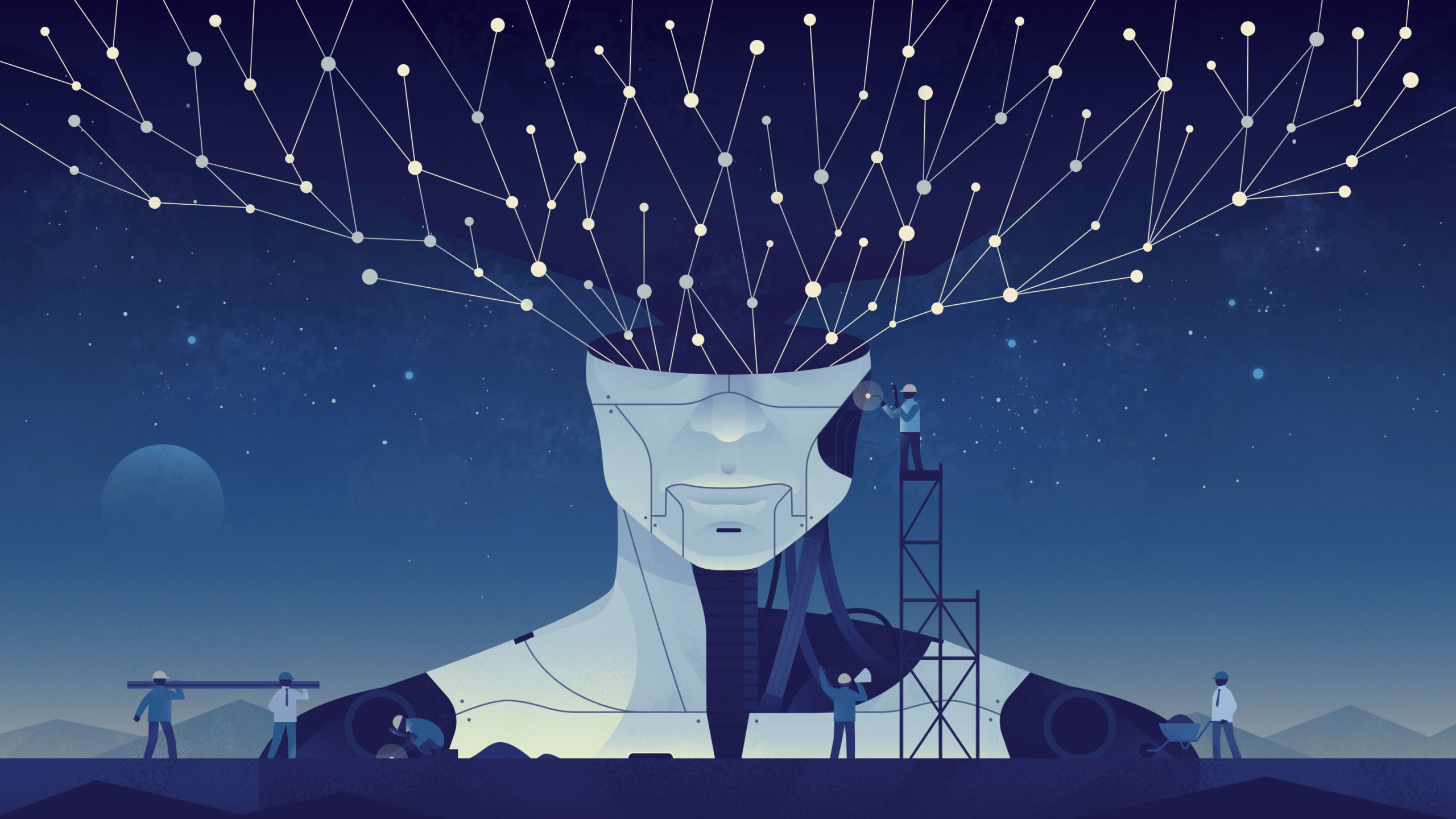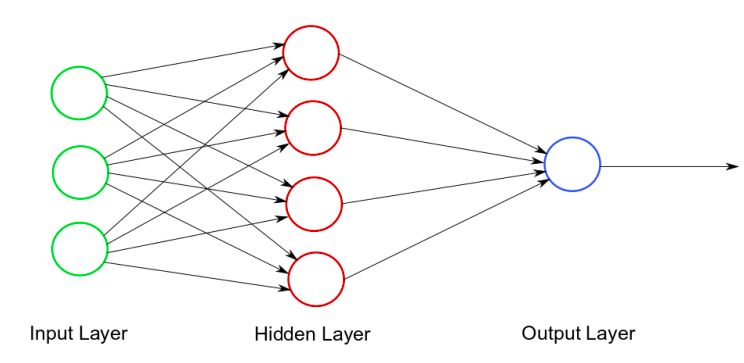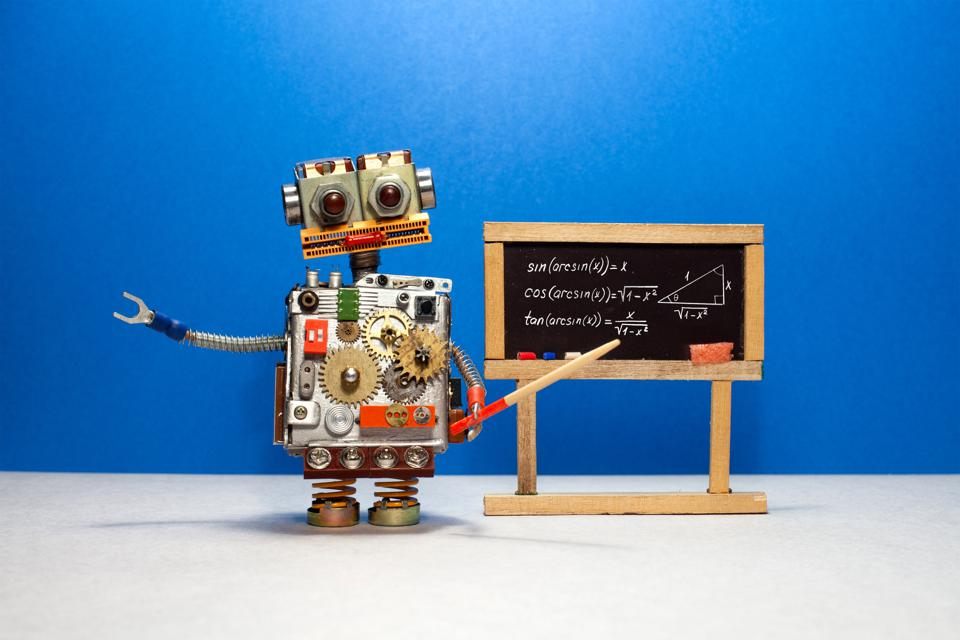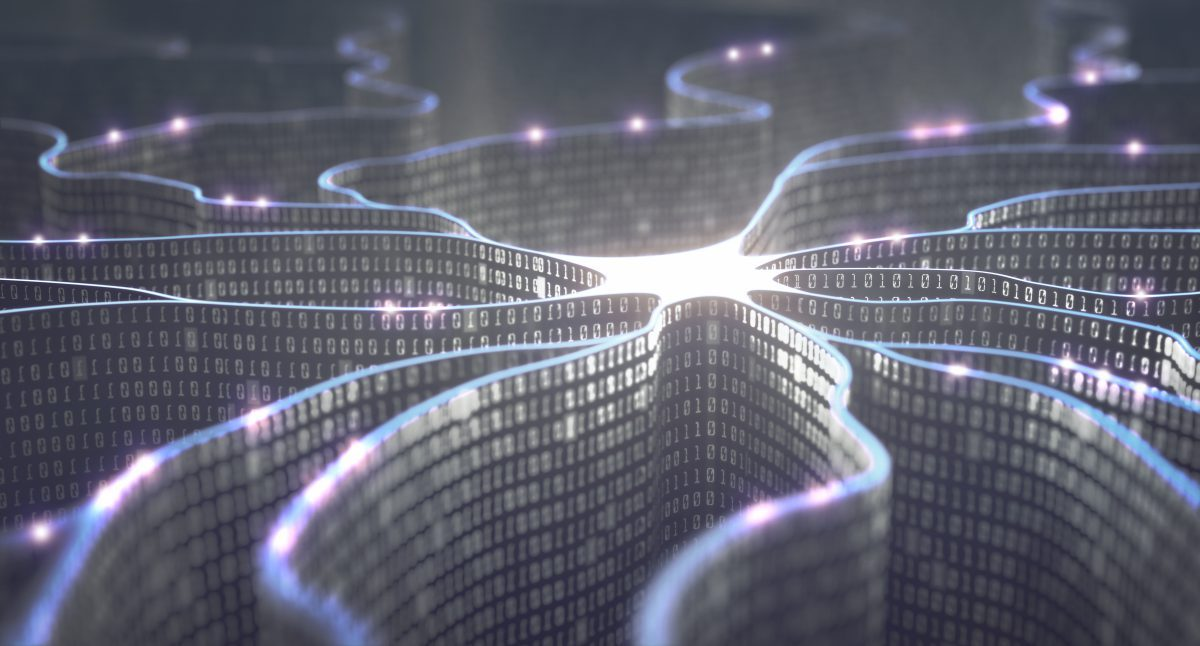# A Beginner’s Guide to Artificial Neural Networks – Part 2

August 26, 2020 - 8 minutes readOver the last decade, artificial intelligence (AI) development has been moving at the speed of light. As a result, chatbots, self-driving cars, and deepfakes have now all become viable. Artificial neural networks are behind many of these advancements.

Welcome to the second part of our special series on artificial neural networks! In our first chapter, we defined what an artificial neural network is and examined its relationship with the natural neural networks inside our brains. In case you missed it, you can check it out here.

In this post, we’ll explore more of how artificial neural networks work and learn. Let’s dive right in!

### The Role of Non-Linearity in Artificial Neural Networks

As we mentioned in the previous entry of this series, neurons are the fundamental element of our brains. Learning, thinking, and imagining are all made possible by the coordinated activation of neurons. This activation function is what initially processes information to determine if the neurons will actually be activated.

If the neuron isn’t activated, nothing happens. While doesn’t sound particularly important, it’s actually crucial to the functioning of a neural network. Without the activation function, the neural network will have to process a bunch of information that has no bearing on the output. Our brains have limited capacity, and this mechanism helps to optimize its use. All of this discussion is meant to demonstrate one central concept of artificial neural networks: non-linearity.Many real-life variables possess non-linear behavior. For example, let’s look at the cost of bananas. For simplicity’s sake, we’ll assume one banana costs \$1. That means that 100 bananas presumably costs \$100. But what if we were to buy 100,000 bananas? It probably wouldn’t cost \$100,000; when it comes to bulk quantities, sellers usually either add expenses of extra packaging to the cost or offer a discount since you’re purchasing a large portion of their inventory.

This is non-linearity in a nutshell. And the activation function of neural networks can use various mathematical principles to determine if non-linearity has been reached and the information should be processed. Let’s define the most common activation function forms:

Binary Step Function: If an input value is above or beneath a specific threshold, the neuron is activated and transmits the same signal to the next layer.

Logistic Function: An ‘S’-shaped curve that’s employed when probabilities are the main criteria used to determine if a neuron should be activated or not. This function’s value always lies between 0 and 1, and it’s generally used when two variables don’t have a linear relationship. You can calculate the slope (the value of a tangent touching the curve at the exact moment when non-linearity is observed) of this curve at any point using a differential function. Logistic functions typically don’t work well for processing data with negative values.

Hyperbolic Tangent Function: This one’s similar to the logistic function, but its value fall between -1 and +1. In other words, it can handle data with negative values.

Rectified Linear Units: The values for this function can be between 0 and positive infinity. The actual function itself works quite simply: Is the input positive? Then you’ll get the value of ‘x’. Any other inputs equal a value of ‘0’. This function is normally used when the relationship between variables is weak and may get excluded by the activation function.Besides activation functions, hidden layers also play a crucial role in neural networks. This layer is located between the input layer and output layer. It’s tasked with refining the processing so that inconsequential variables are eliminated. If a dataset contains a significant number of instances where a change in an input variable’s value affects the output variable substantially, the hidden layer will show this relationship. Essentially, the hidden layer makes it easy for artificial neural networks to pass stronger signals to the next processing layer.

### How Does an Artificial Neural Network Learn?

So now that you know the most common activation functions and understand how the hidden layer functions, you’re probably wondering this: How does an artificial neural network actually learn?

Let’s answer this question with another: What is learning? In the simplest terms possible, learning is the establishment of causality between two things (objects, variables, processes, etc.). This goes for humans, computers, and even machine learning.

It’s worth noting that causality can be tough to establish. For instance, if two variables are moving in the same direction, it’s hard to say which variable is causing the other one to move. As humans, our brains are developed enough that we can often determine causality intuitively. But how do you teach this to a machine? You use a cost function!Mathematically speaking, a cost function is the squared difference between dataset’s actual value and its output value. It gets squared because the difference is sometimes negative. Ideally, the artificial neural network should minimize the cost function to its lowest value possible. This can be achieved by adjusting the network’s weights (remember the synaptic connections we discussed in our previous post?).

For each input-to-output cycle, the objective is to minimize the cost function. Going from input to output is known as forward propagation. Conversely, backward propagation is the process of using your output data to reduce the cost function by adjusting weights in reverse order, from the last hidden layer to the input layer.And there you have it — this is essentially how an artificial neural network learns!

### Stay Tuned for Applications of Artificial Neural Networks

We hope that this brief overview of how artificial neural networks work and learn has activated some of your brain’s neurons! Now you have a more in-depth understanding of how startups and companies in tech hubs like San Francisco, Seattle, and London are using this technology to make new innovations.

Stay tuned for the third and final entry of this series. We’ll discuss artificial neural network applications such as outcome prediction, natural language processing, and self-driving cars more!

Tags: , , , , , , , , , , , , , , , ,